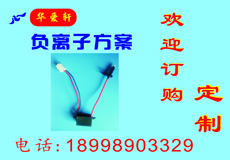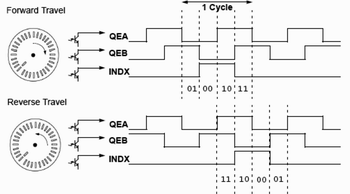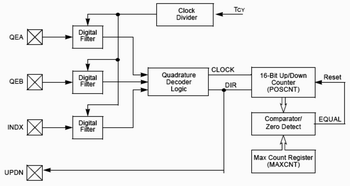dsPIC30F的正交编码器接口（QEI）模块提供了一个与增量光编码器的接口，允许从电机或机械系统中，获得速度和相对转子位置信息。QEI模块提供16位时基，接受从增量编码器连接的A、B和标志信号。电机速度和位置信息能用×2或×4分辨率进行测量。
QEI模块有两个速度通道：A相（QEA）和B相（QEB）。两个通道有对应关系，如果A相超前B相，则认为电机的方向是正转或正向的。如果A相滞后B相，则认为电机的方向是反转或反向的。第三个通道是INDX，称标志脉冲通道，电机转子每旋转一周产生一个脉冲，用于确立转子绝对位置的基准。图1是这三个信号的相对时间关系。

可编程译码器（提供计数器脉冲和计数方向）。
16位上/下位置计数器。
计数方向状态。
×2和×4分辨率计数。
位置计数器复位的两个模式。
普通用途16位定时器/计数器模式。
QEI或计数器事件产生中断。

在0°-360°范围内测量转子角位置。已知增量编码器光栅的数量，设置成相应的定标系数。用一个16位无符号变量表示。
测量角速度。已知的电机最高速度。用一个有符号16位变量表示，这里转子正转时符号是（+），反转时符号是（-）的。

QEI模块初始化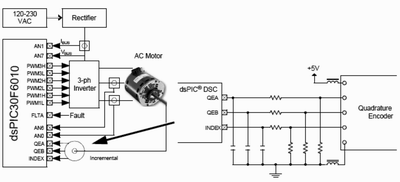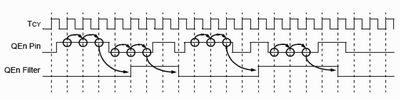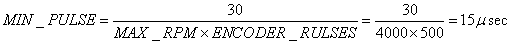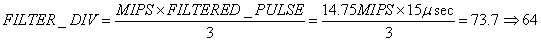void InitQEI（viod）
{
//配置QEI引脚作为数字输入
QEICONbit.QEIM=0; //禁止QEI模块
QEICONbit.CNTERR=0; //清除计数错误
QEICONbit.QEISIDL=0; //睡眠期间连续工作
QEICONbit.SWPAB=0; //QEA和QEB交换
QEICONbit.PCDOUT=0; //标准I/O引脚工作
QEICONbit.POSRES=1;
//标志脉冲复位位置计数器
DELTCONbits.CEID=1; //禁止计数错误中断
DELTCONbits.QEOUT=1;
//允许QEn引脚数字滤波器输出
DELTCONbits.QECK=5;
//对QEn数字滤波器1：64时钟分频
DELTCONbits.INDOUT=1;
// 允许INDEX引脚数字滤波器输出
DELTCONbits.INDCK=5;
//对INDEX数字滤波器1：64时钟分频
POSCNT=0;
//复位位置计数器
QEICONbit.QEIM=6;
//用INDEX位置计数器复位×4模式
Return;
}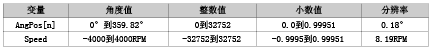MAX_COUNT_PER_REV=PULSES_PER_REV×COUNT_INC_PER_REV-1=500×4-1=1999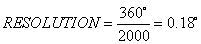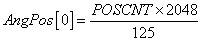int AngPos={0,0};
//用于速度计算的两个变量
int POSCNTcopy=0;
{
POSCNTcopy=(int)POSCNT;
If (POSCNTcopy<0)
POSCNTcopy=- POSCNTcopy;
AngPos= AngPos
AngPos=(unsigned int)(((unsigned long) POSCNTcopy*2048)/125;
//0<=POSCNT<=19991到0<=POSCNT<=32752
return;
}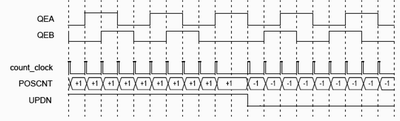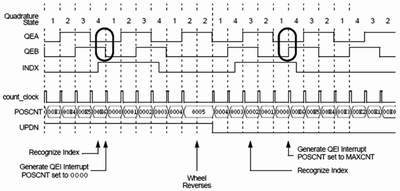void InitTMR1(void)
{
TMR1=0;             //复位定时器计数器
T1CONbit.TON=0;     //关闭定时器1
T1CONbit.TSIDL=0;   //睡眠期间连续工作
T1CONbit.TGATE=0;  //禁止进入精确定时器
T1CONbit.TCS=0;    //使用Tcy作为时钟源
T1CONbit.TCK=2;    //Tcy/64作为输入时钟
PR1=1728 ;
//用64预分频器确定中断周期=0.0075秒
IFSobit.T1IF=0;      //清定时器1中断标志
IECobits.T1IE=1;     //允许定时器1中断
T1CONbits.TON=1;   //打开定时器1
turn;
}

#define  MAX_CNT_PER_REV(500*4-1)
#define MAXSPEED (unsigned int)(((unsigned long)MAX_CNT_PER_REV*2048)/125)
#define HALFMAXSPEED (MAXSPEED>>1)
int Speed;
void_attribute_((_interrupt_))_T1Interrupt(void)
{
IFSobits.T1IF=0;//清定时器1中断标志
PositionCalculation();
Speed=AngPos- AngPos;
If(Speed>=0)
{
if (Speed>=(HALFMAXSPEED)
Speed=Speed-MAXSPEED;
}
else
{
if (Speed<-(HALFMAXSPEED)
Speed=Speed+MAXSPEED;
}
Speed*=2;
Return;
}

如果测量的速度和角位置需要比较高的分辨率，可以用下列方法：

使用输入捕捉通道代替转换每转计数周期测量。
用每转产生更多脉冲地增量计数器。

(郭盛利)

•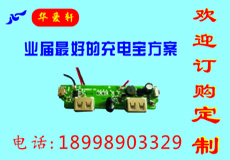•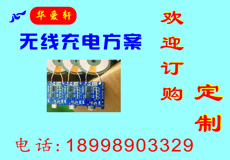•